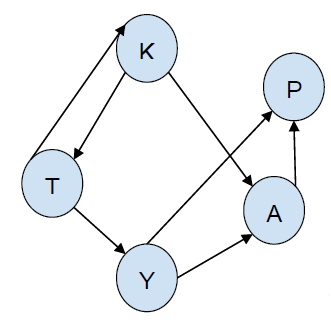# Print all paths from a given source to a destination using BFS in C++

In this problem we are given a directed graph and we have to print all paths from the source to the destination of the graph using Breadth first Search (BFS).

Directed graph is a graph in with edges that are directed from vertex a to b.

Let’s take an example to understand the problem -Source = K destination = P

Output

K -> T -> Y -> A -> P
K -> T -> Y -> P
K -> A -> P

Here, we have found paths from K to P. We have traversed paths and printed all paths from K that direct us to P.

To print all paths from source to destination, we will have to traverse the graph and store paths and then print valid paths.

In the case of using DFS, the process is easy but in this case its a little bit tricky to implement.

To solve this problem, we will need a queue that will store paths. A starting from the source node we will start traversing the array using BFS. traverse

the tree and then check in the queue. If destination vertex is reached then print the queue elements otherwise ignore it.

## Example

The below program will make the solution more clear −

Live Demo

#include <bits/stdc++.h>
using namespace std;
void printPath(vector<char>& path) {
int size = path.size();
for (int i = 0; i < size; i++)
cout<<path[i]<<" ";
cout<<endl;
}
int isVertexVisited(char x, vector<char>& path) {
int size = path.size();
for (int i = 0; i< size; i++)
if (path[i] == x)
return 1;
return 0;
}
void pathSourceToDestination(vector<vector<char> >&g, char src, char dst, int v) {
queue<vector<char> > q;
vector<char> path;
path.push_back(src);
q.push(path);
while (!q.empty()) {
path = q.front();
q.pop();
char last = path[path.size() - 1];
if (last == dst)
printPath(path);
for (int i = 0; i < g[last].size(); i++) {
if (!isVertexVisited(g[last][i], path)) {
vector<char> newpath(path);
newpath.push_back(g[last][i]);
q.push(newpath);
}
}
}
}
int main() {
vector<vector<char> > g;
int v = 4;
g.resize(4);
g['X'].push_back('S');
g['X'].push_back('A');
g['X'].push_back('N');
g['A'].push_back('S');
g['N'].push_back('X');
g['N'].push_back('A');
char src = 'N', dst = 'S';
cout<<"path from src "<<src<<" to dst "<<dst<<" are \n";
pathSourceToDestination(g, src, dst, v);
return 0;
}

## Output

path from src N to dst S are
N X S
N A S
N X A S